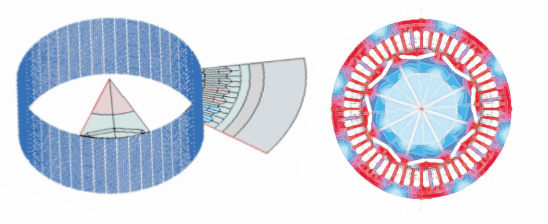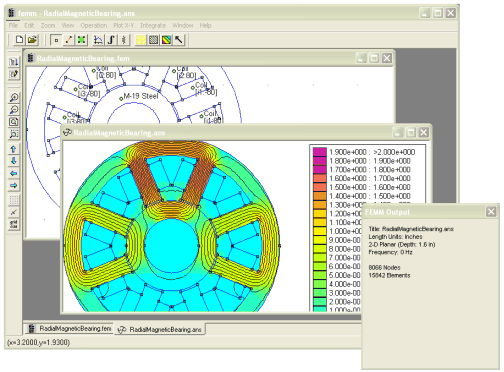Select Page

# Electromagnetic noise / eNVHThe CEVAA simulates and characterizes electromagnetic noise and vibrations of electric motors.

## Our services

### Engineering and testing

• Noise measurements of an electric vehicle (ex: VAE, electric microcar)
• Study of the integration of an electric motor on a chassis
• Determination of reception stiffness and sizing of filtration systems
• Design and production of acoustic hoods to attenuate electromagnetic noise

### Vibroacoustic simulation

• Calculation of electromagnetic forces
• Calculation of the resulting magnetic vibrations
• Calculation of the resulting magnetic noises

### Engine modelling and optimization

• Quick NVH calculation during early electromagnetic design loops
• Optimized NVH calculation in the detailed design phase (coupling with finite element structural analysis) over the entire operating range
• Advanced post-processing for NVH root cause analysis
• Optimization of the design of noise attenuation techniques for electric motors

In addition to electromagnetic noise, the study of electrical machines requires consideration of mechanical, thermal, and vibro-acoustic aspects.## CEVAA test capacities

• Synchronous measurement of noise, vibration and electrical signals
• Motion transmission analysis (command tracking, diagnostics)
• Analysis of transfer pathways to determine injected forces
• Characterization of small and medium sources (direct measurement of forces)
• Benchmark analytical correction methodology to increase the upper limit of analysis frequency

Means and software

• 2D and 3D streams
• Optistruct
• Actran
• 3D laser vibrometryFEMM (Finite element method electromagnetic simulations)

## Application examples

### #1 ELECTROMAGNETIC MODEL

• Radial magnetic force, tangential magnetic force on stator teeth (as a function of time and frequency), magnetic modes.
• Non-linear magnetostatic model by finite elements (FEMM, Flux)

### #2 STRUCTURAL MODEL

• Modal analysis
• Magnetic force wave at a given frequency, eigenfrequencies, operational deformations

### #3 ACOUSTIC MODEL

• Calculation of frequency responses
• Contribution of the modes of the structure
• Optimization with sensitivity study

## OUR TECHNICAL MEANS

High Performance Computing (HPC)